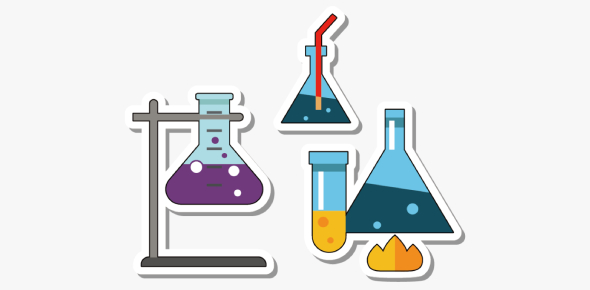# Ultimate Organic Chemistry Test! Quiz

14 Questions | Total Attempts: 280Settings.

• 1.
• A.

Hydrolysis

• B.

Amino Acid

• C.

Dehydration Synthesis

• D.

Nucleic Acid

• 2.
__________________ breaks polymers apart.
• A.

Hydrolysis

• B.

Amino Acid

• C.

Dehydration Synthesis

• D.

Nucleic Acid

• 3.
Which of these are the types of compounds?(There's more than 1)
• A.

Lipids

• B.

Proteins

• C.

Carbohydrates

• D.

Nucleic Acid

• 4.
The monomer of a carbohydrate is ___________________.
• A.

Amino Acid

• B.

Glucose

• C.

Fatty Acid

• D.

Glycerol

• 5.
The monomer of a protein is ___________________.
• A.

Glucose

• B.

Amino Acid

• C.

Fatty Acid & Glycerol

• D.

Nucleotide

• 6.
The monomer of a lipid is ___________________.
• A.

Glucose

• B.

Amino Acid

• C.

Fatty Acid & Glycerol

• D.

Nucleotide

• 7.
The monomer of nucleic acid is ___________________.
• A.

Glucose

• B.

Amino Acid

• C.

Fatty Acid & Glycerol

• D.

Nucleotide

• 8.
A protein catalyst is___________________.
• A.

Polarities

• B.

• C.

Enzyme

• D.

Heat Capacity

• 9.
Carbon is the element of life (it's unique) because ___________________.
• A.

It has a positive and negative end

• B.

It has 7 bonding points

• C.

It has only a negative end

• D.

It has 4 bonding points

• 10.
What makes water a universal solvent?
• A.

It has a positive and negative end

• B.

It has 3 bonding points

• C.

Because its water

• D.

Its' polarities

• 11.
Why is water a polar molecule?
• A.

It has 4 bonding points

• B.

Its polarities

• C.

It has a positive and negative end

• D.

Its heat capacity

• 12.
___________________ is when water is attracted to a solid surface.
• A.

Cohesion

• B.

• C.

Capillary Action

• D.

Surface Tension

• 13.
___________________ is when water is attracted to water.
• A.

Cohesion

• B.

• C.

Capillary Action

• D.

Surface Tension

• 14.
The maximum amount of heat a molecule can have before a phase change is called ___________________.
• A.

Capillary action

• B.

Cohesion

• C.Back to top